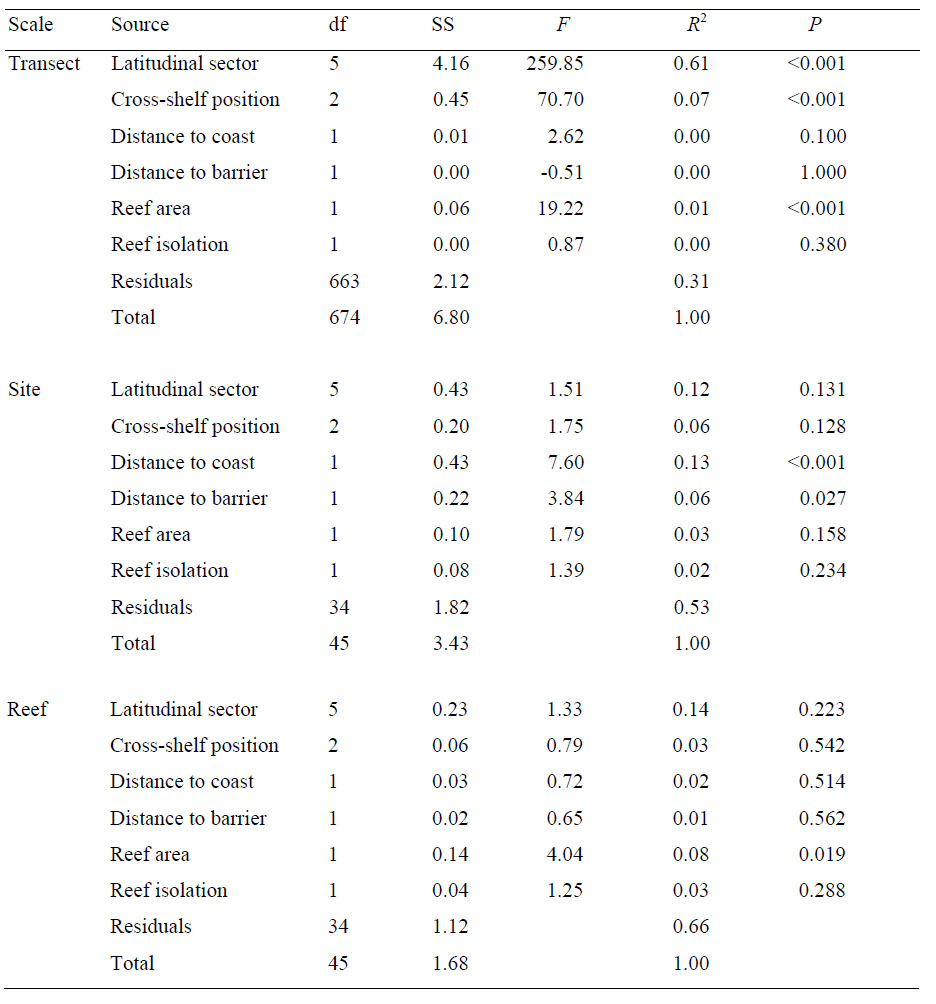### Camille Mellin, Lael Parrott, Serge Andréfouët, Corey J. A. Bradshaw, M. Aaron MacNeil, and M. Julian Caley. 2012. Multi-scale marine biodiversity patterns inferred efficiently from habitat image processing. Ecological Applications 22:792–803.

Appendix A. Variation partitioning in image complexity variables calculated at each spatial scale.

TABLE A1. Variation partitioning in image complexity variables calculated at each spatial scale as given by multivariate analysis of variance using Bray-Curtis distance matrix and 1000 permutations; shown are degrees of freedom (df), sum of squares (SS), F ratio (F), coefficient of determination (R2) and associated probability of committing a Type I error when rejecting the null hypothesis of no effect (P).[Back to A022-043]